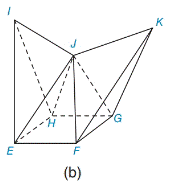Chapter 9.4, Problem 3EElementary Geometry For College St...

7th Edition
Alexander + 2 others
ISBN: 9781337614085

Solutions

Chapter
SectionElementary Geometry For College St...

7th Edition
Alexander + 2 others
ISBN: 9781337614085
Textbook ProblemFor figure (b) of Exercise 1, find the number of faces, vertices, and edges in the polyhedron. Then verify Euler’s equation for that polyhedron.

To determine

To find:

The number of faces, vertices and edges in the polyhedron and verify Euler’s equation.

Explanation

Approach:

A) Polyhedron

1) A polygon is a two dimensional shape form with more than two straight lines.

2) A polyhedron is a three-dimensional solid shape.

3) Each flat surface of a polyhedron is a polygon and is called a face.

4) The line segment where two faces of a polyhedron meet is called an edge.

5) The point where three or more edges of a polyhedron meet is called a vertex.

B) Euler’s Equation

A very important relationship between the number of vertices, faces and edges of solid shapes was discovered by a Swiss mathematician Leonard Euler.

It states V+FE=2.

Where, V=Number of vertices, F=number of faces, E=number of edges.

Calculation:

In the polyhedron EFGHIJK,

The number of vertices (V) =7(E, F, G, H, I, J, K)

Number of faces (F) =9(ΔEFJ,ΔKFG,ΔIEH,ΔJ

Still sussing out bartleby?

Check out a sample textbook solution.

See a sample solution

The Solution to Your Study Problems

Bartleby provides explanations to thousands of textbook problems written by our experts, many with advanced degrees!

Get Started

In Problems 7-12, find the third derivative.

Mathematical Applications for the Management, Life, and Social Sciences

In Exercises 69-74, rationalize the numerator. 69. 2x3

Applied Calculus for the Managerial, Life, and Social Sciences: A Brief Approach

Divide the following numbers. 18.

Contemporary Mathematics for Business & Consumers